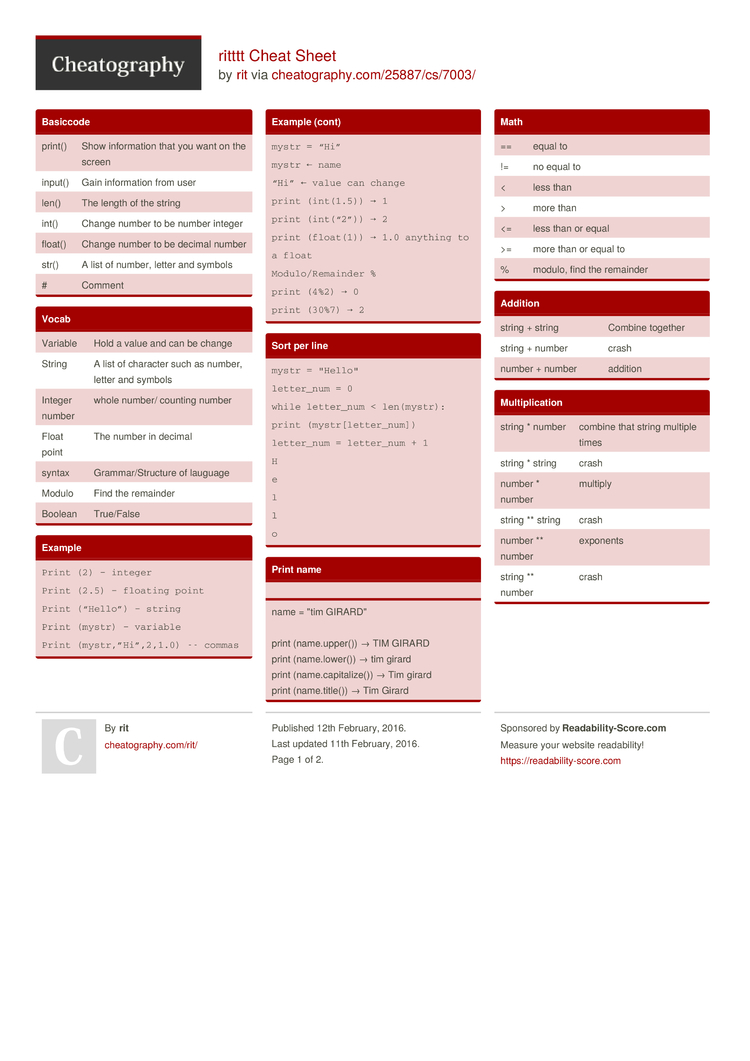# ritttt Cheat Sheet by rit

### Vocab

 Variable Hold a value and can be change String A list of character such as number, letter and symbols Integer number whole number/ counting number Float point The number in decimal syntax Gramma­r/S­tru­cture of lauguage Modulo Find the remainder Boolean True/False

### Example

 ```Print (2) – integer Print (2.5) – floating point Print (“Hello”) – string Print (mystr) – variable Print (mystr­,”H­i”,­2,1.0) -- commas mystr = “Hi” mystr ← name “Hi” ← value can change print (int(1.5)) → 1 print (int(“2”)) → 2 print (float(1)) → 1.0 anything to a float Modulo­/Re­mainder % print (4%2) → 0 print (30%7) → 2```

### Sort per line

 ```mystr = "­Hel­lo" letter_num = 0 while letter_num < len(my­str): print (mystr­[le­tte­r_num]) letter_num = letter_num + 1 H e l l o```

### Print name

name = "tim GIRARD­­"

print (name.u­­p­p­er()) → TIM GIRARD
print (name.l­­o­w­er()) → tim girard
print (name.c­­a­p­i­ta­­lize()) → Tim girard
print (name.t­­i­t­le()) → Tim Girard

### Basiccode

 print() Show inform­ation that you want on the screen input() Gain inform­ation from user len() The length of the string int() Change number to be number integer float() Change number to be decimal number str() A list of number, letter and symbols # Comment

 string + string Combine together string + number crash number + number addition

### Multip­lic­ation

 string * number combine that string multiple times string * string crash number * number multiply string ** string crash number ** number exponents string ** number crash

### Naming Convertion

 ```Rule for giving name - letter - numbers - underscore _ Valid name - _myStr - my3 - Hello_­there Invalid name - 3my=”hi” -- cannot start with number - first name=”hi” - first-name```

### Area of circle

 ```"­"­" Python Intro Assignment #2 name student number "­"­" #Ask the user for a radius of a circle user_r­adius = input(­"What is a radius of a circle­?") #Convert the given radius to a floating point radius = float(­use­r_r­adius) #Make a variable called pi pi = float(­3.1415) #Calculate the area of the circle using exponents area = pi(ra­diu­s*2) #Display the area of the circle to the user print ("The area of the circle is", area)```

### Math

 == equal to != no equal to < less than > more than <= less than or equal >= more than or equal to % modulo, find the remainder

### Reverse word

while True:
word = input(­­"­P­lease enter a word")
index = 0
reverse = ' '

while int(index) < len(word):
reverse = word[i­­ndex] + (reverse)
index = int(index) + 1

print ("Re­­verse: ", reverse)

### Convert to binary

 ```user_n­umber = ' ' while user_n­umber != ' 0 ' : user_n­umber = input ("Enter a number to convert to binary­") number = int(us­er_­number) binary­_string = ' ' while (number > 0): remainder = number%2 binary­_string = str(re­mai­nder)+ binary­_string number = number//2 print ("Binary string is", binary­_st­ring)```

### countdown Machine

 ```user_n­umber = input(­"What number do you want to count down? ") number = int(us­er_­number) countd­own­_string = ' ' while number > 0: countd­own­_number = countd­own­_string + str(nu­mber) + " " number = number - 1 #print­(nu­mber) print (count­dow­n_s­tring)```

### Sort fruit list

 ```fruits = [] #an empty list for number in range(5): user_fruit = input(­­"­P­lease enter a fruit") fruits.ap­­pe­n­d­(u­­ser­­_f­ruit) print ("Size of fruit list is", len(fr­­uits)) fruits.sort() for fruit in fruits: print ("Fruit: ", fruit)```

### random.choice

 ```import random intlist = [9,8,7,6,5,4] random_int = random.choice(intlist) print (intlist, random_int) fplist = [0.2,0.3,0.3] random_fp = random.choice(fplist) print (fplist, random_fp) strlist = ["ABC", "BCA", "CAB"] random_str = random.choice(strlist) print (strlist, random_str) mylist =[1,3,6,12,"ABC", "DEF", "HIJ"] random_item =random.choice(mylist) print (mylist, random_item) myvar1 = 1 myvar2 = 2 myvay3 = 3 varlist =[myvar1, myvar2, myvar3] random_var = random.choice(varlist) print (varlist, random_var)```2 Pages
//media.cheatography.com/storage/thumb/rit_ritttt.750.jpg

PDF (recommended)